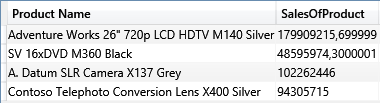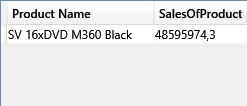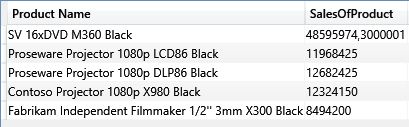Variables are a major feature that makes writing DAX code easier. Moreover, it greatly increases the readability and reusability of your code. Syntax is very simple, as in the following example:

```[Growth %] :=
VAR
CurrentSales = SUM ( Sales[Quantity] )
VAR
SalesLastYear = CALCULATE (
SUM ( Sales[Quantity] ),
SAMEPERIODLASTYEAR ( 'Date'[Date] )
)
RETURN
IF (
AND ( CurrentSales <> 0, SalesLastYear <> 0 ),
DIVIDE (
CurrentSales - SalesLastYear,
SalesLastYear
)
)
```

The VAR keyword introduces the definition of a variable. You can have as many variables as needed in a single expression, and each one has its own VAR definition. The RETURN keyword defines the expression to return as the result. Inside RETURN expression, you can use the variables, which are replaced by the computed value. From the previous example, you can already appreciate the advantage in terms of code readability. By assigning names to expressions, the code is simpler to read and maintain over time. Yet, there is a lot more to learn about variables.

First, variables can contain tables. For example, you can define an expression like the following one:

```[RedSalesLastYear] :=
VAR
RedProducts = FILTER (
ALL ( Product[Color] ),
Product[Color] = "Red"
)
VAR
LastYear = SAMEPERIODLASTYEAR ( 'Date'[Date] )
RETURN
CALCULATE ( SUM ( Sales[Quantity] ), RedProducts, LastYear )
```

In this example, RedProducts and LastYear are tables, not scalar values. You can define variables with both scalar values and tables. There is no difference in the syntax or in their usage.

Another point that might not be obvious is that variables are part of an expression. Wherever you write an expression, you can define a variable. Moreover, DAX evaluates variables in the context of their definition, not in the one where they are used. For example, imagine you want to retrieve the list of all products that sold, individually, more than 1% of your total sales. In standard DAX, before variables, you had to write:

```EVALUATE
FILTER (
VALUES ( Product[Product Name] ),
[SalesAmount]
>= CALCULATE ( [SalesAmount], ALL ( Product ) ) * 0.01
),
"SalesOfProduct", [SalesAmount]
)
```

By leveraging ALL ( Product ) in the inner CALCULATE, you compare the sales of the current product with 1% of the sales of all the products. The result is four products, of different colors:The problem of this formula (and all of the formulas that follow the same pattern) is that it stops working as soon as you put any filter on the product outside of it, e.g. by means of an outer CALCULATETABLE. As an example, the following query returns the black products that sold more than 1% of all products, not the ones that sold more than 1% of black products.

```EVALUATE
CALCULATETABLE (
FILTER (
VALUES ( Product[Product Name] ),
[SalesAmount]
>= CALCULATE ( [SalesAmount], ALL ( Product ) ) * 0.01
),
"SalesOfProduct", [SalesAmount]
),
Product[Color] = "Black"
)
```

The result is a single row, the black one of the previous figure:By using variables, the query becomes much easier to author and works regardless of outer filters:

```EVALUATE
CALCULATETABLE (
VAR
OnePercentOfSales = [SalesAmount] * 0.01
RETURN
FILTER (
VALUES ( Product[Product Name] ),
[SalesAmount] >= OnePercentOfSales
),
"SalesOfProduct", [SalesAmount]
),
Product[Color] = "Black"
)
```

The result is now the expected one: 5 rows of black products.It is worth spending a few minutes reading the formula, because it shows the power of variables very well. The variable OnePercentOfSales is defined inside ADDCOLUMNS. Thus, it is evaluated in the filter context in which ADDCOLUMNS is computed, i.e. that of black products. Thus, OnePercentOfSales is the value of 1% of black products. Once the variable is evaluated, we start iterating the product table and, inside the loop, we evaluate [SalesAmount], which returns the sales of the current product, and OnePercentOfSales, which returns the 1% sales of black products. Because we avoided using ALL and modifying the filter context inside the iteration, this latter formula works no matter what outer filter you set with CALCULATETABLE. Said in other words, variables let you access the outer filter context, which is probably the single most wanted feature of seasoned DAX coders.

You are not limited to use variables in measures or in queries, they work perfectly fine in calculated columns. In fact, once you start using variables, you can completely get rid of the EARLIER function. For example, to count the number of products with a price higher than the current one, you previously had to write a calculated column like this:

```Product[ListPriceRankDense] =
COUNTROWS (
FILTER (
VALUES ( Product[Unit Price] ),
Product[Unit Price] > EARLIER ( Product[Unit Price] )
)
) + 1
```

EARLIER is probably the most hated function in DAX and many people find it hard to use it, maybe because the name should have been OUTER, instead of EARLIER. By using variables, the same expression becomes much clearer and easier to write:

```Product[ListPriceRankDense] =
VAR
CurrentPrice = Product[Unit Price]
RETURN
COUNTROWS (
FILTER (
VALUES ( Product[Unit Price] ),
Product[Unit Price] > CurrentPrice
)
) + 1
```

You can appreciate, even in this expression, that the variable is evaluated outside of FILTER. Thus, it evaluates the price of the current product. When used inside FILTER it already has a defined value. Thus, EARLIER is no longer required in this expression.

Finally, variables lead to the single evaluation of complex subexpression. The DAX optimizer use variables to guarantee that their evaluation happens only once, resulting in much faster code, whenever you had the same subexpression that needs to be evaluated more than once.

ALLCALCULATE modifier

Returns all the rows in a table, or all the values in a column, ignoring any filters that might have been applied.

`ALL ( [<TableNameOrColumnName>] [, <ColumnName> [, <ColumnName> [, … ] ] ] )`

CALCULATEContext transition

Evaluates an expression in a context modified by filters.

`CALCULATE ( <Expression> [, <Filter> [, <Filter> [, … ] ] ] )`

CALCULATETABLEContext transition

Evaluates a table expression in a context modified by filters.

`CALCULATETABLE ( <Table> [, <Filter> [, <Filter> [, … ] ] ] )`

ADDCOLUMNSReturns a table with new columns specified by the DAX expressions.

`ADDCOLUMNS ( <Table>, <Name>, <Expression> [, <Name>, <Expression> [, … ] ] )`

EARLIERReturns the value in the column prior to the specified number of table scans (default is 1).

`EARLIER ( <ColumnName> [, <Number>] )`

FILTERReturns a table that has been filtered.

`FILTER ( <Table>, <FilterExpression> )`